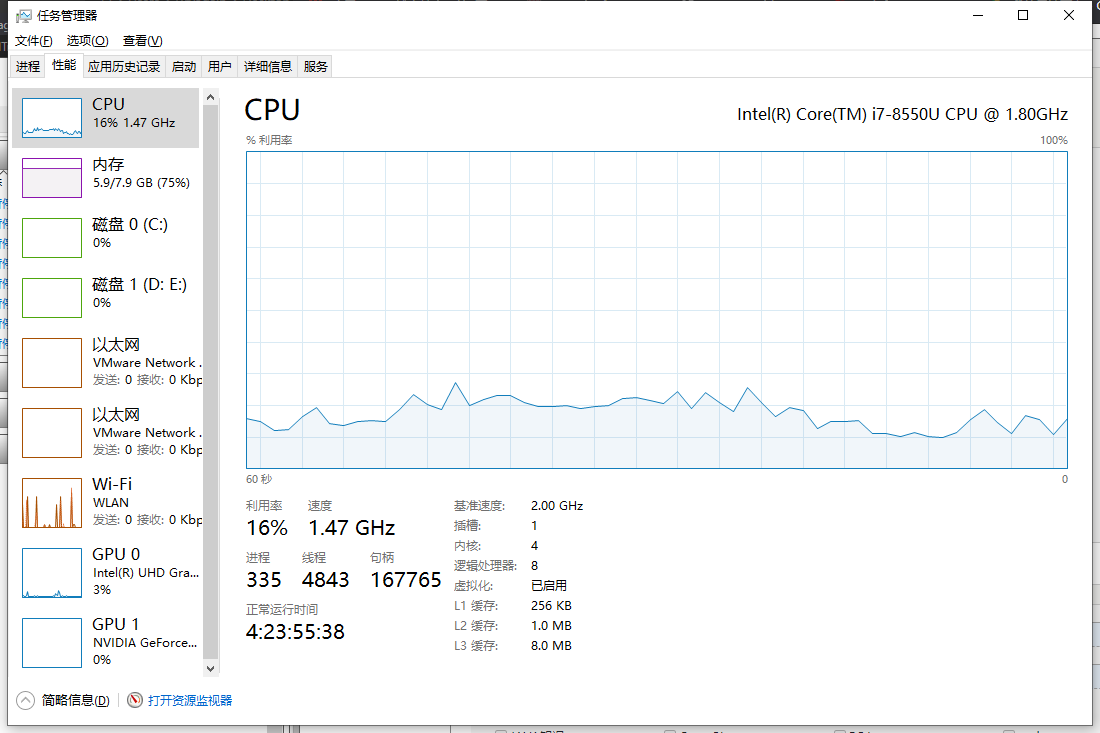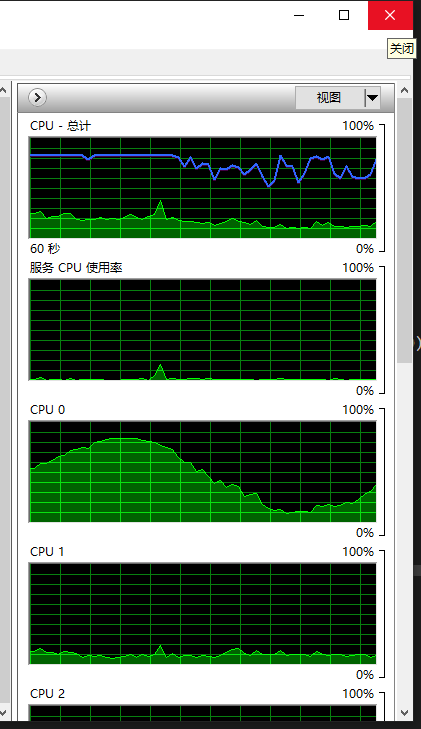2019/04/10 10:10

# 让 CPU 利用率听你指挥

1. CPU 和占用率固定在50%，为一条直线
2. CPU 的占用率为一条直线，具体占用率由命令行参数决定（参数范围 1~100）
3. CPU 的占用率状态是一条正弦曲线

``````while True:
for i in range(7200000):
pass
time.sleep(0.01)``````

``````next:
mov     eax, dword ptr[i]     ; i放入寄存器
mov     dword ptr [i], eax    ;  寄存器赋回i
cmp     eax, dword ptr [i]    ;  比较i和n
j1      next                  ;  i小于n时重复循环``````

``````busyTime = 0.01
while True:
startTime = time.clock()
while((time.clock() - startTime) <= busyTime):
pass
time.sleep(busyTime)``````

``````import time
import mathimport affinity
from multiprocessing import Process, cpu_count

def exec_fun():
SAMPLING_COUNT = 200 # 抽样点数量
PI = math.pi    # pi
TOTAL_AMPLITUDE = 300 # 每个抽样点对应时间片
busySpan = []

amplitude = TOTAL_AMPLITUDE / 2
for i in range(SAMPLING_COUNT):
busySpan.append((amplitude + math.sin(PI * radian) * amplitude) / 1000.0)
# print(busySpan[i], TOTAL_AMPLITUDE - busySpan[i])

j = 0
while True:
startTime = time.clock()
# print(startTime)
while ((time.clock() - startTime) <= busySpan[j]):
pass
# print('sleep')
time.sleep(0.3 - busySpan[j])
j  = (j + 1) % SAMPLING_COUNT

exec_fun()``````这跟说好的好像不太一样啊。是不是因为用的是 python，跑的本来就慢的原因？那试试 C++ 吧

``````#include<stdlib.h>
#include<Windows.h>
#include<math.h>

const int SAMPLING_COUNT = 150;
const double PI = 3.1415926535;
const int TOTAL_AMPLITUDE = 300;

int main()
{
DWORD busySpan[SAMPLING_COUNT];
int amplitude = TOTAL_AMPLITUDE / 2;
double radianIncrement = 2.0 / (double)SAMPLING_COUNT;
for (int i = 0; i < SAMPLING_COUNT; i++) {
busySpan[i] = (DWORD)(amplitude + sin(radian * PI) * amplitude);
printf("%d\t%d\n", busySpan[i], TOTAL_AMPLITUDE - busySpan[i]);
}
DWORD startTime = 0;
for (int j = 0;; j = (j + 1) % SAMPLING_COUNT) {
startTime = GetTickCount();
while ((GetTickCount() - startTime) <= busySpan[j]);
Sleep(TOTAL_AMPLITUDE - busySpan[j]);
}
return 0;
}``````……

……

……

``````if __name__ == "__main__":
p = Process(target=exec_fun)
p.start()
pid = p.pid顺便解决下上面C++的代码，在 main() 函数最开始加入下面代码

``SetThreadAffinityMask(GetCurrentThread(), 1);``

# 小节

0
0 收藏

### 作者的其它热门文章0 评论
0 收藏
0